# PSAT Math : Completing Incomplete Tables

## Example Questions

2 Next →

### Example Question #11 : Use Data To Construct And Interpret A Two Way Table: Ccss.Math.Content.8.Sp.A.4

A teacher at a high school conducted a survey of freshman and found that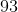students had a curfew and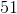of those students were also honor roll students. There werestudents that did not have a curfew, but were on the honor roll. Last, they found thatstudents did not have a curfew nor were on the honor roll. Given this information, how many students were not on the honor roll?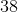Explanation:

To help answer this question, we can construct a two-way table and fill in our known quantities from the question.

The columns of the table will represent the students who have a curfew or do not have a curfew and the rows will contain the students who are on honor roll  or are not on honor roll. The first bit of information that we were given from the question was thatstudents had a curfew; therefore,needs to go in the "curfew" column as the row total. Next, we were told that of those students,were on honor roll; therefore, we need to putin the "curfew" column and in the "honor roll" row. Then, we were told thatstudents do not have a curfew, but were on honor roll, so we need to putin the "no curfew" column and the "honor roll" row. Finally, we were told thatstudents do not have a curfew or were on honor roll, soneeds to go in the "no curfew" column and "no honor roll" row. If done correctly, you should create a table similar to the following: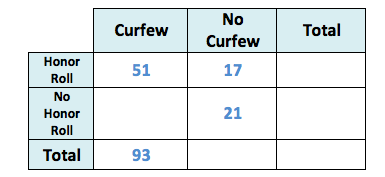Our question asked how many students were not on honor roll. We add up the numbers in the "no honor roll" row to get the total, but first we need to fill in a gap in our table, students who have a curfew, but were not on honor roll. We can take the total number of students that have a curfew,, and subtract the number of students who are on honor roll,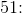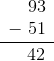This means thatstudents who have a curfew, aren't on honor roll.

Now, we add up the numbers in the "no honor roll" row to get the total: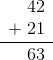This means thatstudents were not on honor roll.

### Example Question #12 : Use Data To Construct And Interpret A Two Way Table: Ccss.Math.Content.8.Sp.A.4

A middle school teacher conducted a survey of the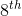grade class and found that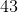students were athletes andof those students drink soda. There werestudents that were not athletes, but drank soda. Last, they found thatstudents were neither athletes nor drank soda. Given this information, how many students don't drink soda?Explanation:

To help answer this question, we can construct a two-way table and fill in our known quantities from the question.

The columns of the table will represent the students who are athletes or are not athletes and the rows will contain the students who drink soda or do not drink soda. The first bit of information that we were given from the question was thatstudents were athletes; therefore,needs to go in the "athlete" column as the row total. Next, we were told that of those students,drinks soda; therefore, we need to putin the "athlete" column and in the "drinks soda" row. Then, we were told thatstudents were not athletes, but drink soda, so we need to putin the "not an athlete" column and the "drinks soda" row. Finally, we were told thatstudents are not athletes or soda drinkers, soneeds to go in the "not an athlete" column and "doesn't drink soda" row. If done correctly, you should create a table similar to the following: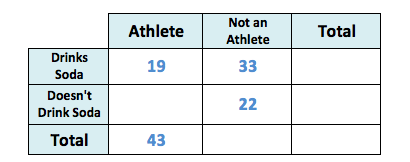Our question asked how many students don't drink soda. We add up the numbers in the "doesn't drink soda" row to get the total, but first we need to fill in a gap in our table, students who were athletes, but don't drink soda. We can take the total number of students who are athletes,, and subtract the number of students who drink soda,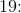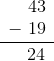This means thatstudents who are athletes, don't drink soda.

Now, we add up the numbers in the "doesn't drink soda" row to get the total: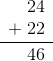This means thatstudents don't drink soda.

2 Next →

### All PSAT Math Resources